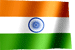RAMESH KRISHNA REDDYOnline Tutorials | PREV | TOP | NEXT

DRONA SERIES
COBOL STUDY MATERIAL

DATE FUNCTIONS

Author : Kishore B.

 ``` DATE FUNCTIONS DATE FUNCTIONS 1. CURRENT-DATE is COBOL intrinsic function to get current date, time and difference between current location time and Greenwich Mean Time. MOVE FUNCTION CURRENT-DATE TO WS-CURRENT-DATE-DATA This function returns a 20-character alphanumeric field in the below format 01 WS-CURRENT-DATE-DATA. 05 WS-CURRENT-DATE. 10 WS-CURRENT-YEAR PIC 9(04). 10 WS-CURRENT-MONTH PIC 9(02). 10 WS-CURRENT-DAY PIC 9(02). 05 WS-CURRENT-TIME. 10 WS-CURRENT-HOURS PIC 9(02). 10 WS-CURRENT-MINUTE PIC 9(02). 10 WS-CURRENT-SECOND PIC 9(02). 10 WS-CURRENT-MILLISECONDS PIC 9(02). 05 WS-DIFF-FROM-GMT PIC S9(04). WS-CURRENT-DATE-DATA contains : 2010111917542857+0800 2. Other COBOL date intrinsic function. a) Converting from Gregorian dates to integer date. COMPUTE integer-date = FUNCTION INTEGER-OF-DATE (Gregorian -date). Gregorian-date must be in form YYYYMMDD. The function result is a 7-digit integer Note: 1600 < YYYY < 9999; 0 < MM < 13; 0 < DD < 32 (provided that day is valid for the specified month and year combination). b) Convert from Integer to Gregorian formats. COMPUTE Gregorian-date = FUNCTION DATE-OF-INTEGER (integer-date) The function result Gregorian-date is an eight-digit integer in the form YYYYMMDD. c) Convert from Julian to Integer formats COMPUTE integer-date = FUNCTION INTEGER-OF-DAY (Julian-date) Julian-date must be in the form YYYYDDD, The function result is a 7-digit integer. Note: 1600 < YYYY < 9999 and 0 < DDD < 367 (provided the day is valid for the specified year) d) Convert from Integer to Julian formats COMPUTE Julian-date = FUNCTION DAY-OF-INTEGER (integer-date) The Julian-date is a seven-digit integer in the form YYYYDDD. Integer-date represents a number of days after December 31, 1600, in the Gregorian calendar. All these functions deal with converting between Gregorian dates or Julian dates and integer format date. This integer format date is number of days from fixed date Example : Converting Gregorian format date 20101202 to Julian format date. COMPUTE integer-date = FUNCTION INTEGER-OF-DATE (Gregorian -date). This converts 20101202(Gregorian date) to 0149720(integer format date). COMPUTE Julian-date = FUNCTION DAY-OF-INTEGER (integer-date). This converts 0149720(integer format date) to 2010336(Julian format date YYYDD) using above date functions, one can get add or subtract days from current date. It is easy with these functions. Example-2: Calculate date after 10 days from Today. MOVE FUNCTION CURRENT-DATE TO WS-CURRENT-DATE-DATA. This move statement moves current timestamp (20-bytes) to WS-CURRENT-DATE-DATA. Since we need only current date, we will take 8 bytes from WS-CURRENT-DATE-DATA. COMPUTE ws-integer = FUNCTION INEGER-OF-DATE (WS-CURRENT-DATE-DATA(1:8)) This converts 20101202 to integer form 0149720 ( i.e. no of days from fixed date) ADD 10 TO ws-integer This calculates the integer form date of future date. it will add 10 days to ws-integer. COMPUTE ws-future-date = FUNCTION DATE-OF-INTEGER (ws-integer). Convert integer form date (i.e. 149730) to date format i.e. 20101222 ```

NEXT CHAPTER TOPIC : COBOL

Visit COBOL books section in this site for good books